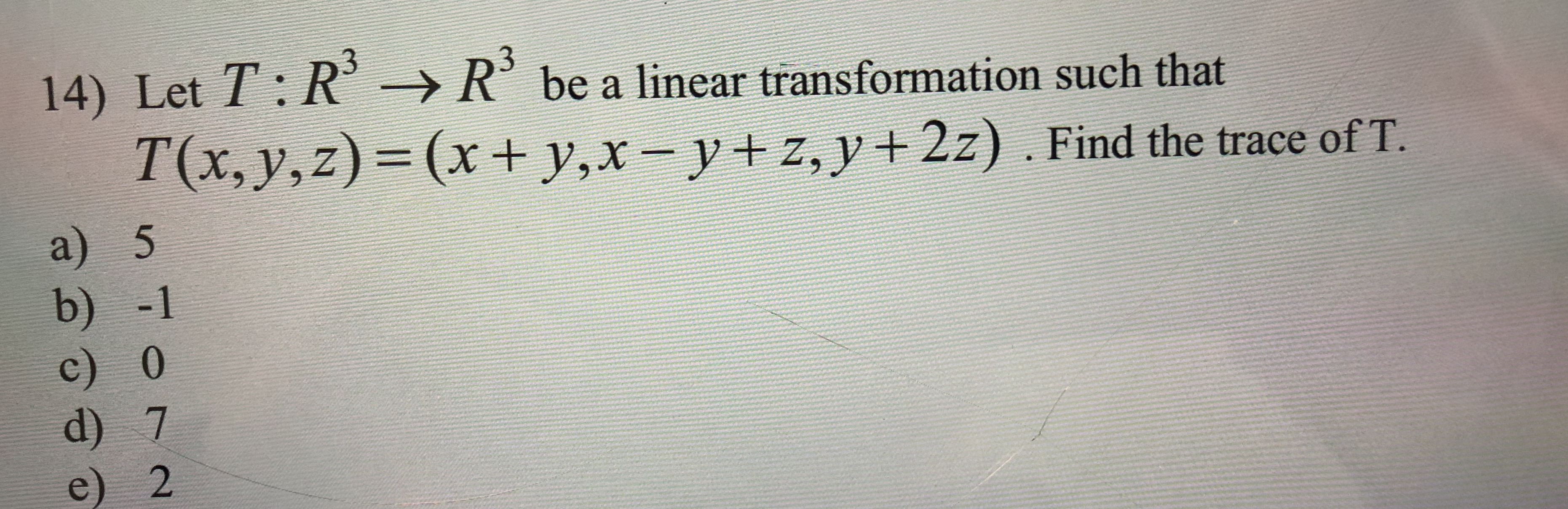# 14) Let T: Rbe a linear transformation such thatRT(x, y,z)= (x + y,x-y+z, y2z) . Find the trace of T.a) 5b) -1c) 0d) 7e) 2

Question
1 viewshelp_outlineImage Transcriptionclose14) Let T: R be a linear transformation such that R T(x, y,z)= (x + y,x- y+z, y2z) . Find the trace of T. a) 5 b) -1 c) 0 d) 7 e) 2 fullscreen
check_circle

Step 1

Let e1 = (1, 0, 0), e2 = (0, 1, 0) and e3 = (0, 0, 1) be the standard basis in R3.

Find T(e1), T(e2) and T(e3) as follows.

Step 2

Thus, the matrix T(x, y, z) can ...

### Want to see the full answer?

See Solution

#### Want to see this answer and more?

Solutions are written by subject experts who are available 24/7. Questions are typically answered within 1 hour.*

See Solution
*Response times may vary by subject and question.
Tagged in

### Math